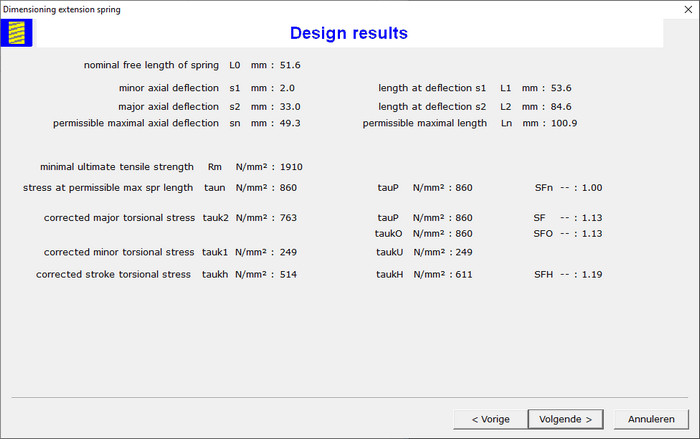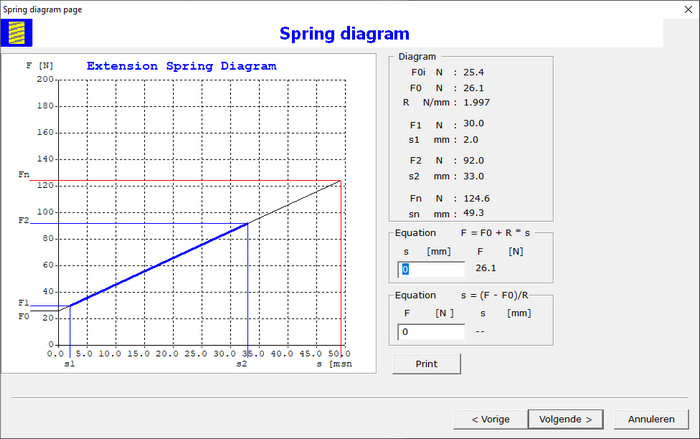The spring properties are determined now and the design calculation results as safety factors on torsional stresses are displayed in wizard page:### Spring Diagram

Spring diagram is displayed in wizard page:The equation of spring line F = F0 + R * s is determined. Get with this equation the axial force F for a given axial extension s. With the inverse equation s = (F - F0)/R the expected extension s is to determine to obtain a given axial load force F. The maximum permissible deflection sn and maximum permissible spring force Fn are determined. The spring diagram is printed with a click of the mouse on the command button Print.

Go to the next page to see the Goodman Diagram for the given number of load cycles.